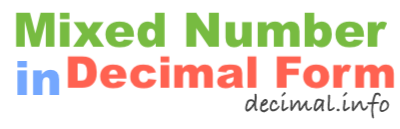What is 1 2/3 in decimal form?To start off, note that 1 2/3 is a mixed number, also known as a mixed fraction. It has a whole number and a fractional number. We have labeled the parts of the mixed number below so it is easier to follow along.

1 = Whole number
2 = Numerator
3 = Denominator

To get 1 2/3 in decimal form, we basically convert the mixed number to a fraction and then we divide the numerator of the fraction by the denominator of the fraction. Here are the detailed math steps we use to convert 1 2/3 mixed number to decimal form:

Step 1: Multiply the whole number by the denominator:

1 × 3 = 3

Step 2: Add the product you got in Step 1 to the numerator:

3 + 2 = 5

Step 3: Divide the sum from Step 2 by the denominator:

5 ÷ 3 = 1.666667

That's it folks! The answer to 1 2/3 in decimal form is displayed below:

1 2/3 ≈ 1.67

Mixed Number in Decimal Form
1 2/3 in decimal form is not all we can do! Here you can convert another mixed number to decimal form.

What is 1 2/4 in decimal form?
Here is the next mixed number on our list that we have converted into decimal form.